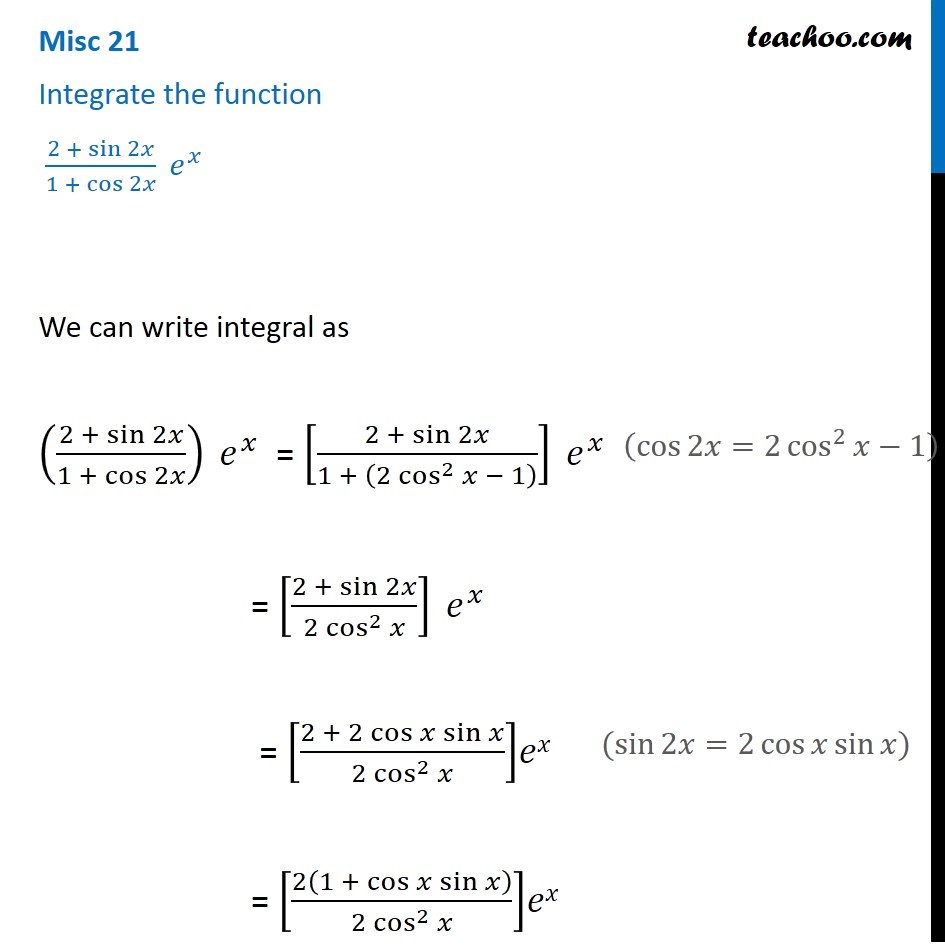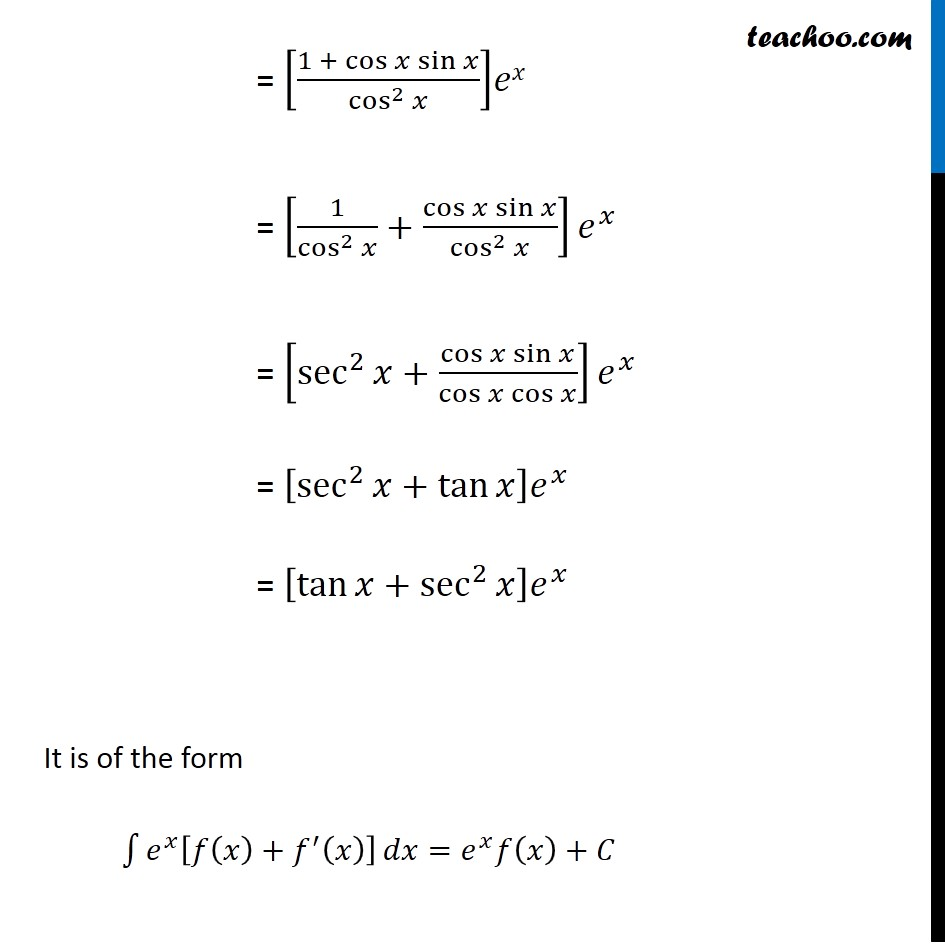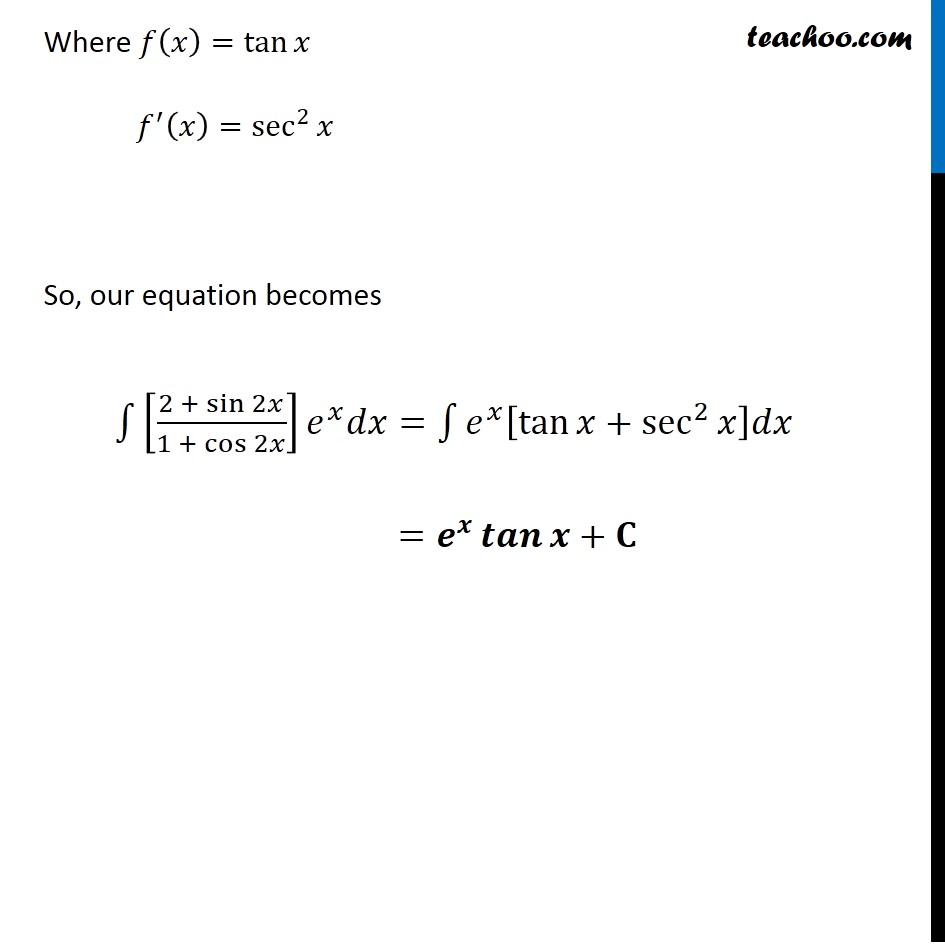1. Chapter 7 Class 12 Integrals (Term 2)
2. Concept wise
3. Integration by parts - e^x integration

Transcript

Misc 21 Integrate the function (2 + sin⁡2𝑥)/(1 + cos⁡2𝑥 ) 𝑒^𝑥 We can write integral as ((2 +〖 sin〗⁡2𝑥)/(1 + cos⁡2𝑥 )) 𝑒^𝑥 = [(2 + sin⁡2𝑥)/(1 + (2 cos^2⁡〖𝑥 − 1〗 ) )] 𝑒^𝑥 = [(2 + sin⁡2𝑥)/(2 cos^2⁡𝑥 )] 𝑒^𝑥 = [(2 + 2 cos⁡〖𝑥 sin⁡𝑥 〗)/(2 cos^2⁡𝑥 )]𝑒𝑥 = [2(1 +〖 cos〗⁡〖𝑥 sin⁡𝑥 〗 )/(2 cos^2⁡𝑥 )]𝑒𝑥 (cos⁡2𝑥=2 cos^2⁡〖𝑥−1〗 ) (sin⁡2𝑥=2 cos⁡〖𝑥 sin⁡𝑥 〗) = [(1 + cos⁡〖𝑥 sin⁡𝑥 〗)/cos^2⁡𝑥 ]𝑒𝑥 = [1/cos^2⁡𝑥 +cos⁡〖𝑥 sin⁡𝑥 〗/cos^2⁡𝑥 ] 𝑒^𝑥 = [sec^2⁡〖𝑥+cos⁡〖𝑥 sin⁡𝑥 〗/cos⁡〖𝑥 cos⁡𝑥 〗 〗 ] 𝑒^𝑥 = [sec^2⁡〖𝑥+tan⁡𝑥 〗 ] 𝑒^𝑥 = [tan⁡〖𝑥+sec^2⁡𝑥 〗 ] 𝑒^𝑥 It is of the form ∫1▒〖𝑒^𝑥 [𝑓(𝑥)+𝑓^′ (𝑥)] 〗 𝑑𝑥=𝑒^𝑥 𝑓(𝑥)+𝐶 Where 𝑓(𝑥)=tan⁡𝑥 𝑓^′ (𝑥)=sec^2⁡𝑥 So, our equation becomes ∫1▒〖[(2 + sin⁡2𝑥)/(1 + cos⁡2𝑥 )] 𝑒^𝑥 𝑑𝑥=∫1▒〖𝑒^𝑥 [tan⁡〖𝑥+sec^2⁡𝑥 〗 ]𝑑𝑥〗〗 =𝒆^𝒙 𝒕𝒂𝒏⁡𝒙+𝐂

Integration by parts - e^x integration

Chapter 7 Class 12 Integrals (Term 2)
Concept wise